Next: Annual equation Up: Lunar motion Previous: Solution of lunar equations

# Variation

Let us, first of all, consider terms in the solution of the lunar equations of motion that depend only on.

According to Equations (11.131) and (11.143),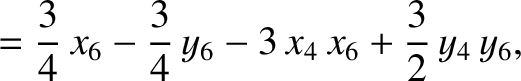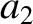(11.215) and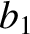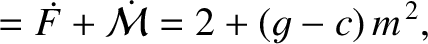(11.216)

It follows from Equations (11.192), (11.193), and (11.197) that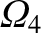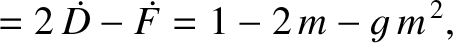(11.217) and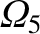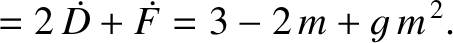(11.218)

According to Equations (11.132) and (11.144),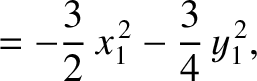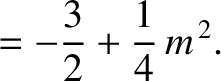(11.219) and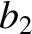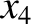(11.220)

where use has been made of the previous expressions for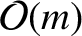and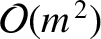. It follows from Equations (11.192), (11.193), and (11.198) that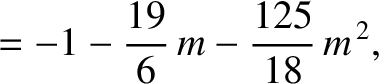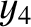(11.221) and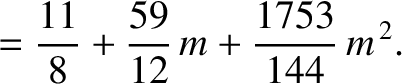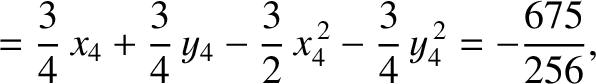(11.222)

Finally, according to Equation (11.125),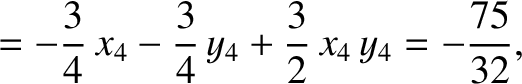(11.223)

where use has been made of the previous expressions forand. Equation (11.189) yields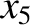(11.224)

It follows from Equations (11.122)-(11.124), (11.157), (11.163), (11.164), (11.175), and (11.176), as well as the previous expressions for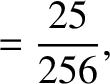,,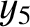,, and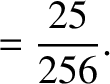, that the net perturbation of the lunar orbit due to terms in the solution of the lunar equations of motion that depend only onis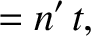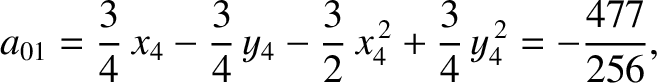(11.225)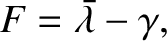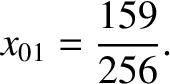(11.226) and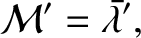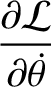(11.227)

The previous expressions are accurate to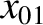.

The first term on the right-hand side of Equation (11.226) is known as variation, and is clearly due to the perturbing influence of the Sun (because it depends only on the parameter, which is a measure of this influence). Variation attains its maximal amplitude around the so-called octant points, at which the Moon's disk is either one-quarter or three-quarters illuminated (i.e., when,,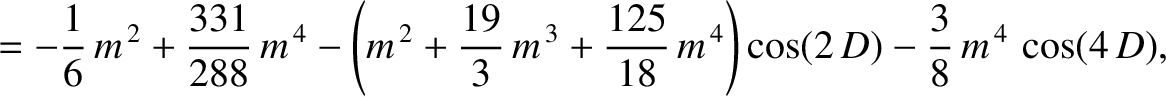, or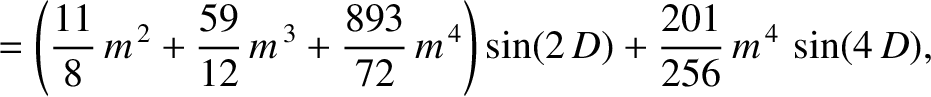). Conversely, the amplitude of variation is zero around the so-called quadrant points, at which the Moon's disk is either fully illuminated, half illuminated, or not illuminated at all (i.e., when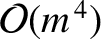,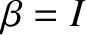,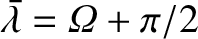, or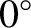). Variation generates a perturbation in the lunar ecliptic longitude that oscillates sinusoidally with a period of half a synodic month.11.2 This oscillation period is in good agreement with observations. However, according to Equation (11.226), the amplitude of the oscillation (calculated using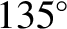) is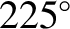arc seconds, which is somewhat less than the observed amplitude of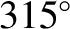arc seconds (Chapront-Touzé and Chapront 1988). This discrepancy between theory and observation is due to the fact that, for the sake of simplicity, our expression for variation only includes contributions that are fourth order, or less, in the small parameter.

Equations (11.103) and (11.225) imply that the mean radius of the lunar orbit is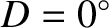(11.228)

In other words,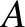is the effective major radius of the orbit. Likewise, Equations (11.97), (11.104), and (11.226) suggest that the mean orbital angular velocity of the Moon is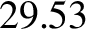(11.229)

Hence, making use of Equation (11.31), we deduce that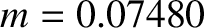(11.230)

Here,is the mass of the Earth, and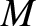the mass of the Moon. The previous expression is accurate to. Equation (11.230) implies that, when applied to the geocentric lunar orbit, Kepler's third law of orbital motion (see Sections 4.7 and 4.16) is slightly modified by the perturbing influence of the Sun (which is parameterized by).Next: Annual equation Up: Lunar motion Previous: Solution of lunar equations
Richard Fitzpatrick 2016-03-31